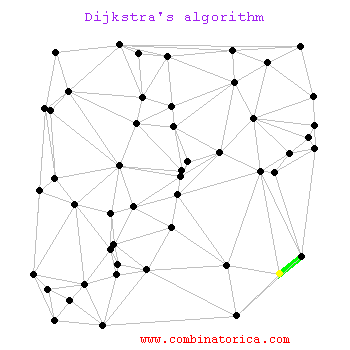## Dijkstra's Algorithm

Dijkstra's Algorithm solves the single-source shortest path problem in weighted graphs. Here we show it running on a planar graph whose edge weights are proportional to the distance between the vertices in the drawing -- thus the weight of an edge is equal to its visible length.

Dijkstra's algorithm starts from a source node, and in each iteration adds another vertex to the shortest-path spanning tree. This vertex is the point closest to the root which is still outside the tree. Watch as the tree grows by radiating out from the root. Note that it is not a breadth-first search; we do not care about the number of edges on the tree path, only the sum of their weights.